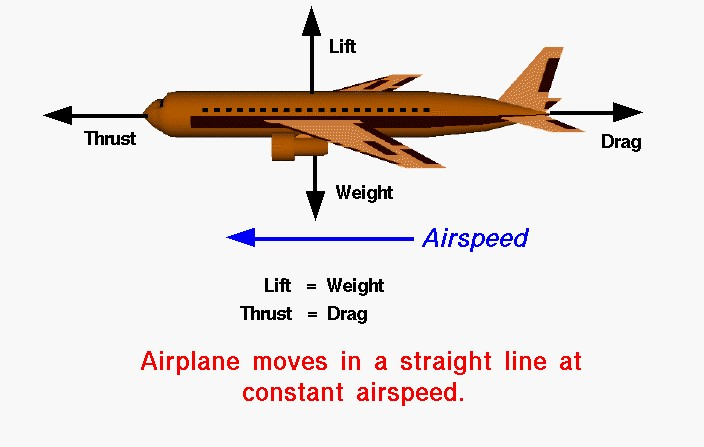# Airplane Cruise – Balanced Forces## Four Forces

There are four forces that act on an aircraft in flight: lift, weight, thrust, and drag. A force is a vector quantity which means that it has both a magnitude (size) and a direction associated with it. If the size and direction of the forces acting on an object are exactly balanced, then there is no net force acting on the object and the object is said to be in equilibrium. From Newton’s first law of motion, we know that an object at rest will stay at rest, and an object in motion (constant velocity) will stay in motion unless acted on by an external force. If there is no net external force, the object will maintain a constant velocity.

## Cruise Velocity

In an ideal situation, the forces acting on an aircraft in flight can produce no net external force. In this situation the lift is equal to the weight, and the thrust is equal to the drag. The closest example of this condition is a cruising airliner. While the weight decreases due to fuel burned, the change is very small relative to the total aircraft weight. The aircraft maintains a constant airspeed called the cruise velocity.

## Relative Velocity

If we take into account the relative velocity of the wind, we can determine the ground speed of a cruising aircraft. The ground speed is equal to the airspeed plus the wind speed using vector addition. The motion of the aircraft is a pure translation. With a constant ground speed, it is relatively easy to determine the aircraft range, the distance the airplane can fly with a given load of fuel.

## Force

If the pilot changes the throttle setting, or increases the wing angle of attack, the forces become unbalanced. The aircraft will move in the direction of the greater force, and we can compute acceleration of the aircraft from Newton’s second law of motion.

Provide feedback